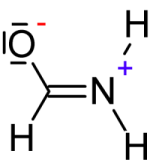Search:

# Detailed study of mesomerism

## (1) Charges and Lewis structures

### Reminder: Lewis structures of atoms and monoatomic ions

Lewis structures represent the last layer of an atom or ion occupied by electrons :If it has in its last layer the same number of electrons than in its Lewis structure, the atom is neutral, if it has n electrons more (less) , it becomes a negatively (positively) charged ion having n negative (positive) charges, by example $O^{2 -}$ has $6 + 2 = 8\;e^-$ in its last layer.

### The formal charges of the atoms in the Lewis structures of molecules or ions

To each atom, we attribute: - Two electrons for each of its own doublets. - An electron for each covalent bond. Comparing then with the Lewis structure of the neutral aton, its charge is deduced, for example:- To each $H$ atom is assigned $1\;e^-$ (one of the covalent bond it forms), thus the formal charge is equal to $0$ whenever - To the $C$ atom are assigned $4\;e^-$ (those that form 4 covalent bonds ), thus the formal charge is equal to $0$ - To the $O$ atom are assigned $7\; e^-$ (3 own doublets and one electron of the covalent bond ), so the formal charge is $-1$ - To the $N$ atom are assigned $4\; e^-$ (one for each of the covalent bonds), thus the formal charge is equal to $1$

Often for reasons of convenience, the own doublets are not represented, for example:- Obviously the $O$ atom above has two doublets and forms two covalent bonds, therefore its formal charge is equal to $0$ - Obviously the $O$ atom below has three doublets and forms one covalent bond, and therefore its formal charge is $-1$ - The central $C$ atom forms four covalent bonds, so its formal charge is $0$ - The lower $C$ atom forms four covalent bonds (one shown, the other three with $H$ atoms), therefore its formal charge is equal to $0$